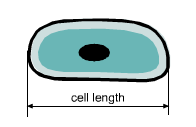Ch 5. Curve Sketching Multimedia Engineering Math CurveSketching Graphing
 Chapter 1. Limits 2. Derivatives I 3. Derivatives II 4. Mean Value 5. Curve Sketching 6. Integrals 7. Inverse Functions 8. Integration Tech. 9. Integrate App. 10. Parametric Eqs. 11. Polar Coord. 12. Series Appendix Basic Math Units Search eBooks Dynamics Fluids Math Mechanics Statics Thermodynamics Author(s): Hengzhong Wen Chean Chin Ngo Meirong Huang Kurt Gramoll ©Kurt GramollMATHEMATICS - CASE STUDY IntroductionPlant Cells Man-made Auxin, a growth chamical, can accelerate the growth speed of plant cells. The cell elongation speed can be modeled with a mathematic expression. To better understand the relationship between the elongation speed and time, the mathematic expression can be plotted. What is known: The mathematics relationship between the promoted elongation speed and the time is       f(t) = -t3 + 5t2 - 3t + 5 The life cycle of Auxin is 7 weeks The unit for elongation speed and time is μm/week and week, respectively. Questions Sketch the curve of the function and show all key features. Approach Find the domain and intercepts of the function. Analysis the symmetric, asymptote, monotonocity, local extreme value, concavity and inflection points of the function.